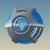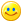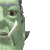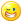## Welcome to the Second Life Forums Archive

These forums are CLOSED. Please visit the new forums HERE

# Vector to... integer! And back!

Cross Quartermass
Registered UserJoin date: 8 Feb 2005
Posts: 6
10-17-2005 11:23
Due to the urging of one of my friends (hi Harris!), I've decided to post a pair of functions that converts a position vector to an integer and back. As the script notes, this is useful anywhere you'd like to stuff a vector into an integer, particularly at object rez. The function's domain is +- 255 x and y, and +- 2047 z. It has a 1 meter resolution.

The comments assume that the reader knows how to use bitwise operations, but not necessarily how to usefully apply them. This should also be a good example script if you're interested in learning about bitwise ops.

CODE
`// PositionVectorToInt, and PositionIntToVector// By Cross Quartermass//// This function compresses a vector representing xyz coordinates into// a 32 bit integer. This is most useful for any place you need to// pass a coord vector but have only an integer to work with, notably// when rezzing an object. The function can represent 511m through// -511m for x and y, and 2047m through -2047m in z. This should// be more than adequate for most purposes in SL.integer PositionVectorToInt(vector input){    integer output; // Our final output variable. We'll concatenate                    // the bitstrings for each vector component then                    // the inverse function will decode the integer.                        float component;// Working variable to deal with each vector                    // component individually.                            integer bitstring;  // Working variable to store the bitstrings to                        // be concatenated.                            integer sign;   // To store the sign of the int.        // I don't know how SL represents signed integers internally,     // be it one or two's complement, so I do a little reconstruction      // here.        component = input.x;        if(component < 0) {        sign = 1; // represents negative sign    }        else {        sign = 0; // represents nonnegative sign    }        component = llFabs(component); // switch to absolute value;    bitstring = (integer)llRound(component); // now we have the integer value    bitstring = (bitstring << 1) | sign; // shift bitstring over a bit                                         // and add the sign to it                                             // Now our bitstring looks like this, for the number 133:        //                  0 1 0 0 0 0 1 1 1 0    //                  \               / |    //                      value       sign        output = bitstring; // so now output has x.        // Same for y so far.    component = input.y;        if(component < 0) {        sign = 1;     }        else {        sign = 0;     }        component = llFabs(component);     bitstring = (integer)llRound(component);     bitstring = (bitstring << 1) | sign;        // Ok, this is different.        output = output << 10; // Shifts output over 10 bits to make room to                          // add the y bitstring.    output = output | bitstring; // This fills in the empty bits left                                  // with y.                                     // For x = 133, y = -27 we now have:        //      0 1 0 0 0 0 1 1 1 0 0 0 0 0 1 1 0 1 1 1    //      \               / | \               / |    //            x value   xsign     yvalue     ysign            // Now, for z.        component = input.z;        if(component < 0) {        sign = 1;     }        else {        sign = 0;     }        component = llFabs(component);     bitstring = (integer)llRound(component);     bitstring = (bitstring << 1) | sign;        // Note that z I'm allowing to be 12 bits long since in SL z    // height is less restricted than xy values. 10 bits for x +     // 10 bits for y + 12 bits for z = 32 bit integer.        output = output << 12;        output = output | bitstring;        // For x = 133, y = -27, and z = 1328 we get        //   0 1 0 0 0 0 1 1 1 0 0 0 0 0 1 1 0 1 1 1 1 0 1 0 0 1 1 0 0 0 0    //   \               / | \               / | \                 / |    //       x value      xsign   y value     ysign   z value        zsign        return output;}    vector PositionIntToVector(integer input){    vector output; // Our final output vector    integer sign; // represents the sign of our component;    float component; // Working variable for the individual components    integer bitmask; // We AND this with the input to get the                     // individual component bitstrings back                         sign = input & 1; // the lowest digit represents sign        // Here we stop using sign as a boolean and instead change it to     // be used for multiplication    if(sign == 0) {        sign = 1;    }        else {        sign = -1;    }        input = input >> 1; // Dumps the first bit, now the first 11 bits                        // are our z value                            bitmask = (1 << 11) - 1; // This produces a bitstring composed                                // of all 1's that is 11 bits long.                                    component = (float)(input & bitmask) * (float)sign; // bitmask cuts away all the                                          // bits not part of z                                              output.z = component; // And the z component is done.        input = input >> 11; // Dump the z value bits        // Ok, now for y.        sign = input & 1;    if(sign == 0) {        sign = 1;    }        else {        sign = -1;    }        input = input >> 1;                             bitmask = (1 << 9) - 1; // 10 here since x and y are 10 bits                                // long.                                    component = (float)(input & bitmask) * (float)sign;                                               output.y = component; // Y is done.        input = input >> 9; // Dump y value bits.        // Finally, x        sign = input & 1;    if(sign == 0) {        sign = 1;    }        else {        sign = -1;    }        input = input >> 1;                             // Since x is the same bit length as y we don't need to reset    // the bitmask.                                    component = (float)(input & bitmask) * (float)sign;                                               output.x = component; // X is done.        // We're done with the input integer, no need to bother dumping    // the x bits.        return output;}// Here's a quick example that shows the functions in action.default{    touch_start(integer detected)    {        vector position = <13.2, -28.4, 232.7>;        llSay(0, "Position vector before processing: " + (string)position);        integer processedInt = PositionVectorToInt(position);        llSay(0, "Position vector in integer form: " + (string)processedInt);        vector processedVector = PositionIntToVector(processedInt);        llSay(0, "Position vector converted back to vector: " + (string)processedVector);    }}`

Note that it does NOT do any sort of error checking. Values beyond the range wrap around, so 256 goes to zero, 257 to 1, etc. I decided not to include error checking, leaving it to the user's discretion how to deal with it. The function could be modified without too much trouble to deal with color vectors as well, if there's interest in that I might post it.

I tested it on a variety of values, in the middle and near the bounds, and found no bugs. However, it hasn't been exhaustively checked, so if there are any bugs please let me knowNada Epoch
The LibrarianJoin date: 4 Nov 2002
Posts: 1,423
Discussion Thread
10-17-2005 19:24
/54/9f/66245/1.html
_____________________
i've got nothing.